# 1.- Introducction¶

This tutorial gives a basic introduction on how to analyze and process a detected gravitational wave, in this case GW150914 , with a Rudin-Osher-Fatemi (ROF hereafter) algorithm. We will employ the algorithm in two different ways: using a unique ROF filter, and using an iteration of filters.

This tutorial is based on the LIGO Open Science Center (LOSC) tutorial for signal processing with GW150914 open data: https://losc.ligo.org/events/GW150914/.

# 2.- Required content¶

Previous to the analysis it is necessary that the user downloads the avaiable data collected by the Advanced LIGO detectors in Hanford and Livingston. The data are accessible at the LOSC’s website. Furthermore, the python libraries numpy, scipy, matplotlib, and h5py must be downloaded and installed, as well as a LOSC script called readligo.py, for reading the data. Now, we have the required tools to start.

In :
import numpy as np
from scipy import signal
from scipy.interpolate import interp1d
from scipy.signal import butter, filtfilt, iirdesign, zpk2tf, freqz

import matplotlib.pyplot as plt
import matplotlib.mlab as mlab
import h5py



# 3.- Single-step ROF algorithm.¶

The first step is to load the data from both signals, Hanford (H1) and Livingston (L1), with the help of readligo.py:

In :
# Handford
fn_H1 = 'H-H1_LOSC_4_V1-1126259446-32.hdf5'
strain_H1, time_H1, chan_dict_H1 = rl.loaddata(fn_H1, 'H1')
# Livingston
fn_L1 = 'L-L1_LOSC_4_V1-1126259446-32.hdf5'
strain_L1, time_L1, chan_dict_L1 = rl.loaddata(fn_L1, 'L1')

fs = 4096
time=time_H1
dt=time-time

# Template
NRtime, NR_H1 = np.genfromtxt('GW150914_4_NR_waveform.txt').transpose()


The analysis of the signal with the single-step ROF method proceeds along the following stages: first, the data are filtered through a band-pass filter which removes part of the experimental noise at high frequencies; second, the ROF algorithm is applied to the data (see below); finally, an additional band-pass filter is emplyed to remove the remaining noise.

We note that the first band-passing stage differs from the second one, since it is indeed a band-passing+notching. This filtering method consists in detecting the known spectral lines (e.g. frequency of the normal modes of vibration of the suspended mirrors, frequency of the electric network, etc) to remove them from the data. This method is applied by using the following functions.

In :
def iir_bandstops(fstops, fs, order=4):
"""ellip notch filter
fstops is a list of entries of the form [frequency (Hz), df, df2]
where df is the pass width and df2 is the stop width (narrower
than the pass width). Use caution if passing more than one freq at a time,
because the filter response might behave in ways you don't expect.
"""
nyq = 0.5 * fs

# Zeros zd, poles pd, and gain kd for the digital filter
zd = np.array([])
pd = np.array([])
kd = 1

# Notches
for fstopData in fstops:
fstop = fstopData
df = fstopData
df2 = fstopData
low = (fstop - df) / nyq
high = (fstop + df) / nyq
low2 = (fstop - df2) / nyq
high2 = (fstop + df2) / nyq
z, p, k = iirdesign([low,high], [low2,high2], gpass=1, gstop=6,
ftype='ellip', output='zpk')
zd = np.append(zd,z)
pd = np.append(pd,p)

# Set gain to one at 100 Hz...better not notch there
bPrelim,aPrelim = zpk2tf(zd, pd, 1)
outFreq, outg0 = freqz(bPrelim, aPrelim, 100/nyq)

# Return the numerator and denominator of the digital filter
b,a = zpk2tf(zd,pd,k)
return b, a

def get_filter_coefs(fs):

# assemble the filter b,a coefficients:
coefs = []

# bandpass filter parameters
lowcut=43
highcut=2047
order = 4

# bandpass filter coefficients
nyq = 0.5*fs
low = lowcut / nyq
high = highcut / nyq
bb, ab = butter(order, [low, high], btype='band')
coefs.append((bb,ab))

# Frequencies of notches at known instrumental spectral line frequencies.
# You can see these lines in the ASD above, so it is straightforward to make this list.
notchesAbsolute = np.array(
[14.0,34.70, 35.30, 35.90, 36.70, 37.30, 40.95, 60.00,
120.00, 179.99, 304.99, 331.49, 510.02, 1009.99])

# notch filter coefficients:
for notchf in notchesAbsolute:
bn, an = iir_bandstops(np.array([[notchf,1,0.1]]), fs, order=4)
coefs.append((bn,an))

# Manually do a wider notch filter around 510 Hz etc.
bn, an = iir_bandstops(np.array([[510,200,20]]), fs, order=4)
coefs.append((bn, an))

# also notch out the forest of lines around 331.5 Hz
bn, an = iir_bandstops(np.array([[331.5,10,1]]), fs, order=4)
coefs.append((bn, an))

return coefs

# Find the coefficients
coefs = get_filter_coefs(fs)

def filter_data(data_in,coefs):
data = data_in.copy()
for coef in coefs:
b,a = coef
# filtfilt applies a linear filter twice, once forward and once backwards.
# The combined filter has linear phase.
data = filtfilt(b, a, data)
return data


Hence, if we implement this process on the data we obtain the following result.

In :
strain_H1_filt=filter_data(strain_H1, coefs)
strain_L1_filt=filter_data(strain_L1, coefs)
# It is necessary to make a shift in L1
strain_L1_shift = -np.roll(strain_L1_filt,int(0.008*fs))

tevent=1126259462.422
plt.figure()
plt.plot(time-tevent,strain_H1_filt,'r',label='H1 strain')
plt.plot(time-tevent,strain_L1_shift,'g',label='L1 strain')
plt.xlabel('time (s) since ')
plt.ylabel('strain')
plt.legend(loc='lower left')
plt.title('Bandpassing+notching filtering')
plt.show()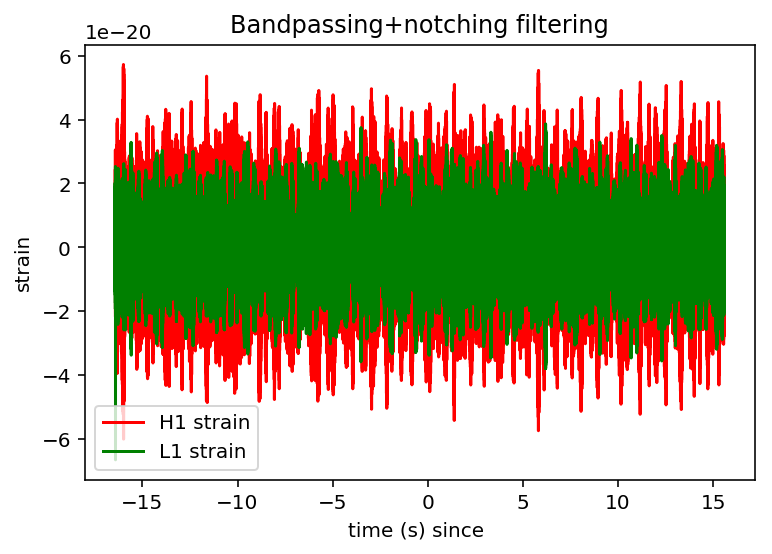As the previous figure shows, this step has removed a large quantity of noise, but it is impossible to discern the existence of the wave. Hence, we need to employ next the ROF filtering.

For an introduction to total-variation techniques, the reader is addressed to  and . For our purpose it is sufficient to know that the ROF algorithm employs a regularization therm with a Lagrange multiplier (µ), whose value indicates the relative importance of the so-called fidelity term. In practice, using a high value of µ means that hardly no noise will be removed from the data, whereas a small value of the regularizatio parameter means that important information could be removed from the signal. Therefore, it is important to first obtain the optimal value of µ.

If we apply the ROF filter to our data, as well as to our template, for µ=0.2 (as mentioned in Torres-Forné et al (2016)) we will be able to observe the shape of gravitational-wave signal GW150914, although it remains still surrounded by noise.

In :
strain_H1_TV=gs1(strain_H1_filt,1,1,0.2,0.01)
strain_L1_TV=gs1(strain_L1_filt,1,1,0.2,0.01)
strain_L1_shift = -np.roll(strain_L1_TV,int(0.008*fs))

# The same steps with the template
strain_NR_filt=filter_data(NR_H1, coefs)
strain_NR_TV=gs1(strain_NR_filt,1,1,0.2,0.01)

plt.figure()
plt.plot(time-tevent,strain_H1_TV,'r',label='H1 strain')
plt.plot(time-tevent,strain_L1_shift,'g',label='L1 strain')
plt.plot(NRtime+0.002,strain_NR_TV,'k',label='NR strain')
plt.xlim([-0.15,0.05])
plt.ylim([-1e-21,1e-21])
plt.xlabel('time (s) since ')
plt.ylabel('strain')
plt.legend(loc='lower left')
plt.title('ROF filter')
plt.show()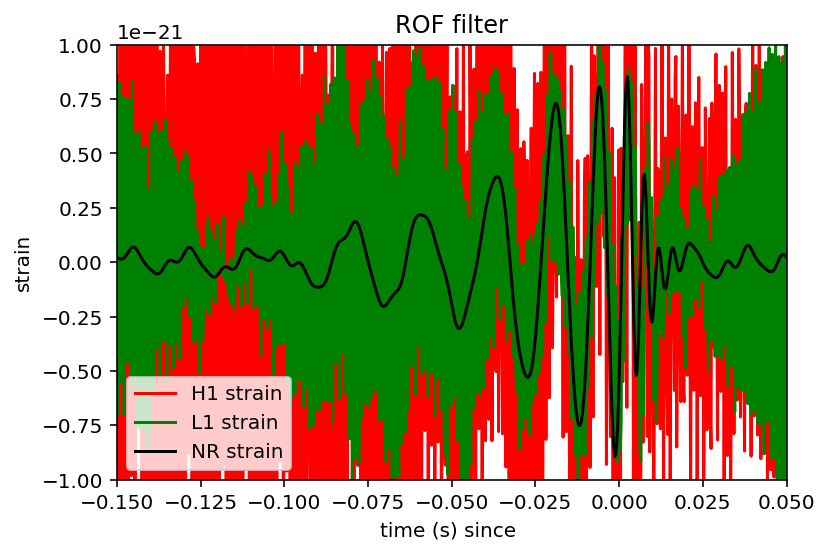Finally, the only step remaining is the implementation of an additional band-pass filter, that can be found in the Pyhton library scipy.signal.

In :
# Fourier transform
NFFT = 1*fs
fmin = 10
fmax = 2000
Pxx_H1, freqs = mlab.psd(strain_H1, Fs = fs, NFFT = NFFT)
Pxx_L1, freqs = mlab.psd(strain_L1, Fs = fs, NFFT = NFFT)
psd_H1 = interp1d(freqs, Pxx_H1)
psd_L1 = interp1d(freqs, Pxx_L1)
bb, ab = butter (4, [20.*2./fs, 300.*2./fs], btype='band')

# Filter application
strain_H1_TV1 = filtfilt(bb, ab, strain_H1_TV)
strain_L1_TV1 = filtfilt(bb, ab, strain_L1_TV)
strain_L1_shift = -np.roll(strain_L1_TV1,int(0.008*fs))
strain_NR_TV1 = filtfilt(bb, ab, strain_NR_TV)

# Plot
tevent=1126259462.422
plt.figure()
plt.plot(time-tevent,strain_H1_TV1,'r',label='H1 strain')
plt.plot(time-tevent,strain_L1_shift,'g',label='L1 strain')
plt.plot(NRtime+0.002,strain_NR_TV1,'k',label='NR strain')
plt.xlim([-0.15,0.05])
plt.ylim([-1e-21,1e-21])
plt.xlabel('time (s) since ')
plt.ylabel('strain')
plt.legend(loc='lower left')
plt.title('Total Variation denoising')
plt.show()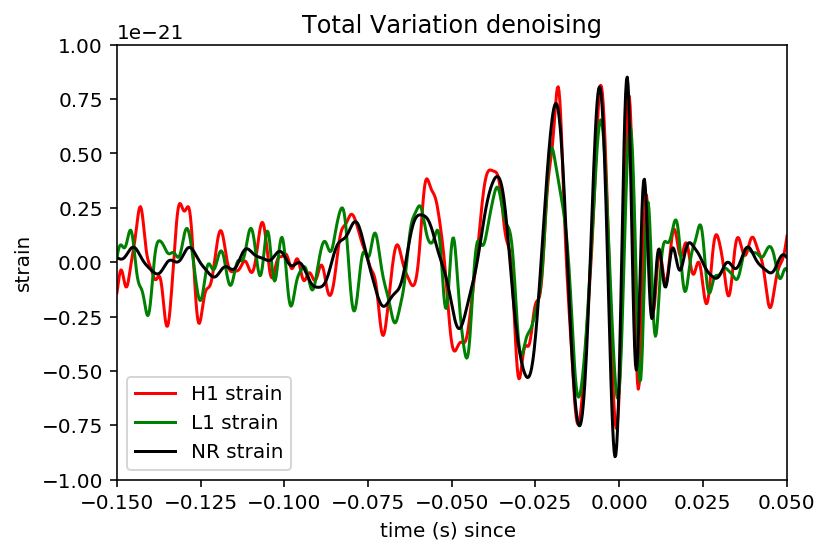If we want to check the spectrogram:

In :
tevent=1126259462.422
deltat=10 # seconds around the event
indxt = np.where((time_H1 >= tevent-deltat) & (time_H1 < tevent+deltat))
# pick a shorter FTT time interval, like 1/8 of a second:
NFFT=fs//8  #fs= 4096 s
NOVL = NFFT*15//16
# and choose a window that minimizes "spectral leakage"
window = np.blackman(NFFT)

# Color
spec_cmap='inferno'

# H1 Spectrogram
plt.figure()
spec_H1, freqs, bins, im = plt.specgram(strain_H1_TV1[indxt], NFFT=NFFT, Fs=fs, window=window,
noverlap=NOVL, cmap=spec_cmap, xextent=[-deltat,deltat], vmin=-550, vmax=-440)
plt.xlabel('time (s) since '+str(tevent))
plt.ylabel('Frequency (Hz)')
plt.colorbar()
plt.axis([-0.5, 0.5, 0, 500])
plt.title('aLIGO H1 strain data near GW150914')

# L1 Spectrogram
plt.figure()
spec_H1, freqs, bins, im = plt.specgram(strain_L1_TV1[indxt], NFFT=NFFT, Fs=fs, window=window,
noverlap=NOVL, cmap=spec_cmap, xextent=[-deltat,deltat], vmin=-550, vmax=-440)
plt.xlabel('time (s) since '+str(tevent))
plt.ylabel('Frequency (Hz)')
plt.colorbar()
plt.axis([-0.5, 0.5, 0, 500])
plt.title('aLIGO L1 strain data near GW150914')
plt.show()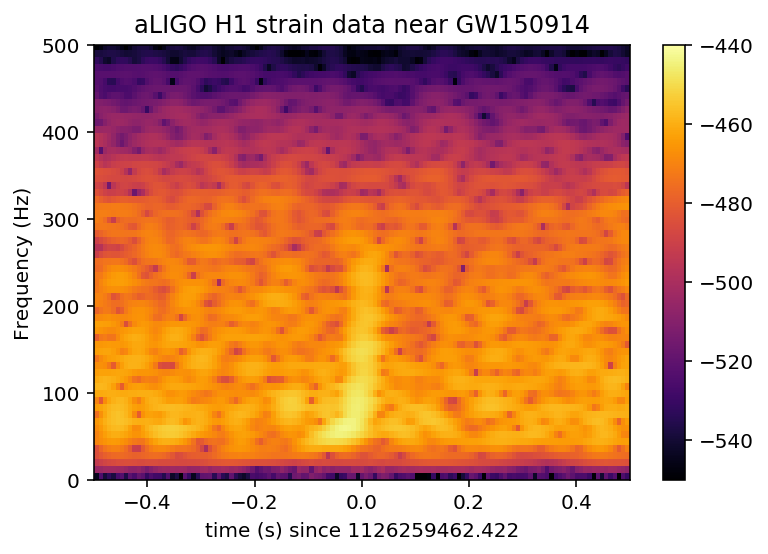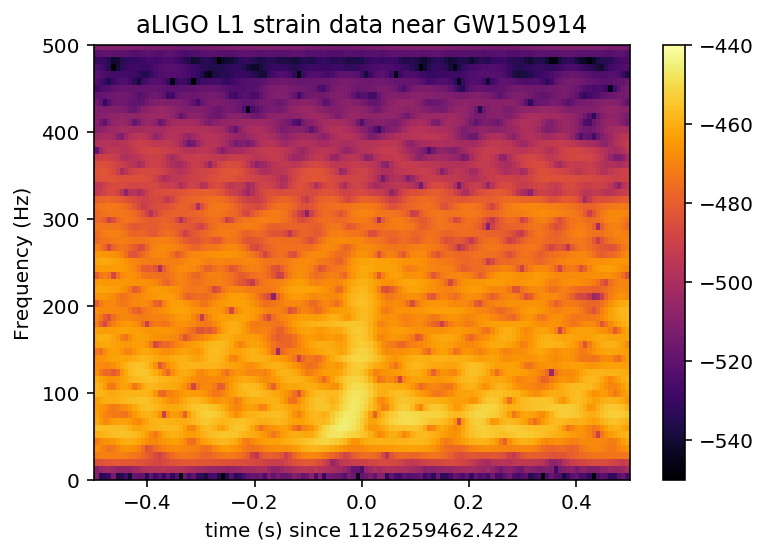# 4.- Iterations of ROF filter¶

This second procedure begins in exactly the same way as the previous one, that is, the data is first filtered with a band-passing+notching filter and then the data are treated with the ROF algorithm. Contrary to the previous procedure, we now iterate the ROF algorithm a sufficient number of times to obtain accurate enough denoised data so that applying an additional band-pass filter becomes unnecessary.

We must now use a large value of the Lagrange multiplier. This is due to the fact that if we used the (optimal) value µ=0.2 for the single-step ROF and we filtered the data more than once, we would end up losing information of the signal. Therefore, we increase the value of the Lagrange multiplier, and expect that the iterative ROF will remove noise gradually.

In :
#  Time-domain filtering - Bandpassing+notching
coefs = get_filter_coefs(fs)

iterations=10 # Number of iterations

# H1
strain_H1_filt=filter_data(strain_H1, coefs)
strain_H1_TV=gs1(strain_H1_filt,1,1,2,0.01)
i=0
while i<iterations:
strain_H1_TV=gs1(strain_H1_TV,1,1,2,0.01)
i+=1

# L1
strain_L1_filt=filter_data(strain_L1, coefs)
strain_L1_TV=gs1(strain_L1_filt,1,1,2,0.01)
i=0
while i<iterations:
strain_L1_TV=gs1(strain_L1_TV,1,1,2,0.01)
i+=1
strain_L1_shift = -np.roll(strain_L1_TV,int(0.008*fs))

# Template
strain_NR_filt=filter_data(NR_H1, coefs)
strain_NR_TV=gs1(strain_NR_filt,1,1,0.2,0.01)
strain_NR_TV1 = filtfilt(bb, ab, strain_NR_TV)

# Plot
tevent=1126259462.422
plt.figure()
plt.plot(time-tevent,strain_H1_TV,'r',label='H1 strain')
plt.plot(time-tevent,strain_L1_shift,'g',label='L1 strain')
plt.plot(NRtime+0.002,strain_NR_TV,'k',label='NR strain')
plt.xlim([-0.15,0.05])
plt.ylim([-1e-21,1e-21])
plt.xlabel('time (s) since ')
plt.ylabel('strain')
plt.legend(loc='lower left')
plt.title('Total Variation denoising with iterations')
plt.show()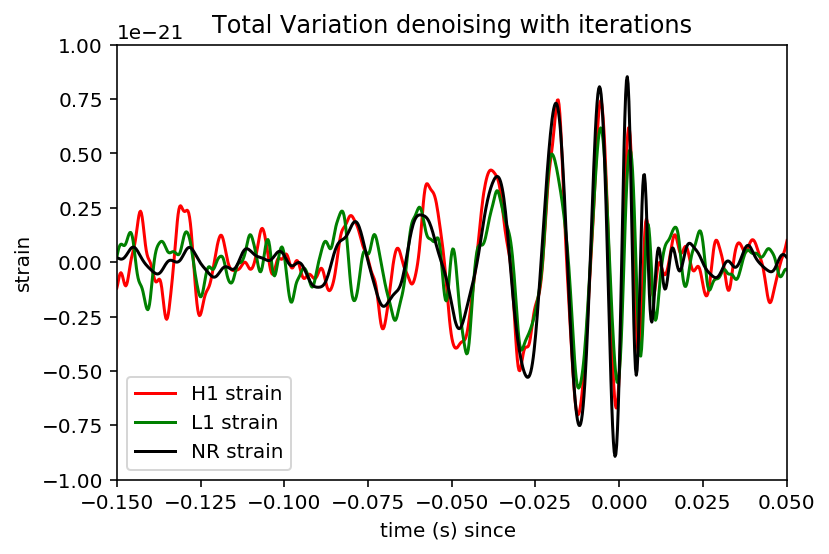If we want to observe the effect of each iteración of the ROF filter, we can display the gradient, where the intesity of the colour blue is associated with the number of iterations.

In :
#  Time-domain filtering - Bandpassing+notching
coefs = get_filter_coefs(fs)
strain_H1_filt=filter_data(strain_H1, coefs)
strain_H1_TV1=gs1(strain_H1_filt,1,1,2,0.01)
strain_H1_TV2=gs1(strain_H1_TV1,1,1,2,0.01)
strain_H1_TV3=gs1(strain_H1_TV2,1,1,2,0.01)
strain_H1_TV4=gs1(strain_H1_TV3,1,1,2,0.01)
strain_H1_TV5=gs1(strain_H1_TV4,1,1,2,0.01)
strain_H1_TV6=gs1(strain_H1_TV5,1,1,2,0.01)
strain_H1_TV7=gs1(strain_H1_TV6,1,1,2,0.01)
strain_H1_TV8=gs1(strain_H1_TV7,1,1,2,0.01)
strain_H1_TV9=gs1(strain_H1_TV8,1,1,2,0.01)
strain_H1_TV10=gs1(strain_H1_TV9,1,1,2,0.01)

# Plot
tevent=1126259462.422
plt.figure()
plt.plot(time-tevent,strain_H1_TV1,label='H1 strain',color='#d4daff')
plt.plot(time-tevent,strain_H1_TV2,label='H1 strain',color='#bac4ff')
plt.plot(time-tevent,strain_H1_TV3,label='H1 strain',color='#a1aeff')
plt.plot(time-tevent,strain_H1_TV4,label='H1 strain',color='#8798ff')
plt.plot(time-tevent,strain_H1_TV5,label='H1 strain',color='#6e82ff')
plt.plot(time-tevent,strain_H1_TV6,label='H1 strain',color='#546dff')
plt.plot(time-tevent,strain_H1_TV7,label='H1 strain',color='#3b57ff')
plt.plot(time-tevent,strain_H1_TV8,label='H1 strain',color='#2141ff')
plt.plot(time-tevent,strain_H1_TV9,label='H1 strain',color='#082bff')
plt.plot(time-tevent,strain_H1_TV10,'#2c328c',label='H1 strain')
plt.xlim([-0.15,0.05])
plt.ylim([-1e-21,1e-21])
plt.xlabel('time (s) since ')
plt.ylabel('strain')
plt.title('ROF iterations')
plt.show()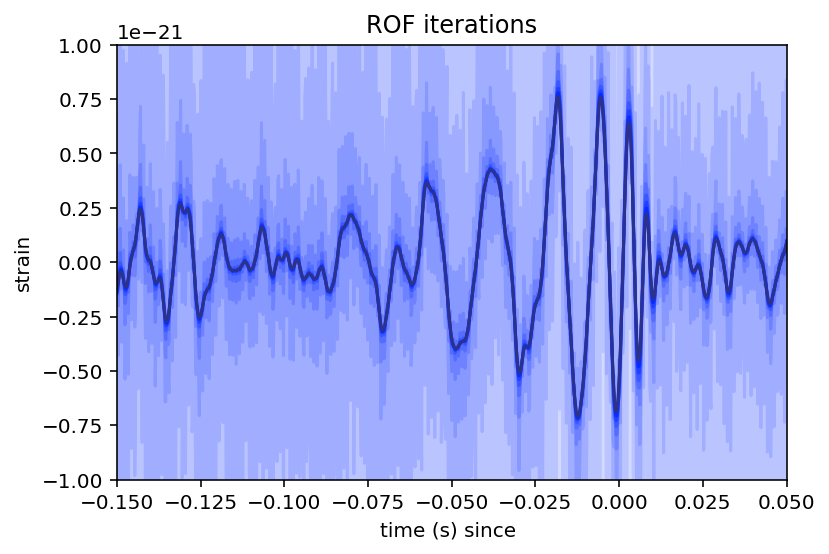# 1.- Introducción¶

In this part of the tutorial, we will denoise the second gravitational wave detected, GW151226 , using the ROF algorithm. As for the case of GW150914, the reading of the data and the band-passing step are based on the LOSC tutorial; https://losc.ligo.org/s/events/gw151226/losc_event_tutorial_gw151226.html

The reading of the detected signal GW161226, as well as the numerical relativity template, will be presented as they are in the LOSC tutorial. The data can be downloaded from the previous link.

In :
#-- SET ME   Tutorial should work with most binary black hole events
#-- Default is no event selection; you MUST select one to proceed.
eventname = ''
# eventname = 'GW150914'
eventname = 'GW151226'
# eventname = 'LVT151012'

# want plots?
make_plots = 1
plottype = "png"
#plottype = "pdf"

In :
#-- SET ME   Tutorial should work with most binary black hole events
#-- Default is no event selection; you MUST select one to proceed.
eventname = ''
# eventname = 'GW150914'
eventname = 'GW151226'
# eventname = 'LVT151012'

# want plots?
make_plots = 1
plottype = "png"
#plottype = "pdf"

In :
# Standard python numerical analysis imports:
import numpy as np
from scipy import signal
from scipy.interpolate import interp1d
from scipy.signal import butter, filtfilt, iirdesign, zpk2tf, freqz
import h5py
import json

# the IPython magic below must be commented out in the .py file, since it doesn't work there.
%matplotlib inline
%config InlineBackend.figure_format = 'retina'
import matplotlib.pyplot as plt
import matplotlib.mlab as mlab

# you might get a matplotlib warning here; you can ignore it.

In :
# Read the event properties from a local json file
fnjson = "BBH_events_v2.json"
try:
except IOError:
print("Cannot find resource file "+fnjson)
print("Quitting.")
quit()

# did the user select the eventname ?
try:
events[eventname]
except:
print('You must select an eventname that is in '+fnjson+'! Quitting.')
quit()

In :
# Extract the parameters for the desired event:
event = events[eventname]
fn_H1 = event['fn_H1']              # File name for H1 data
fn_L1 = event['fn_L1']              # File name for L1 data
fn_template = event['fn_template']  # File name for template waveform
fs = event['fs']                    # Set sampling rate
tevent = event['tevent']            # Set approximate event GPS time
fband = event['fband']              # frequency band for bandpassing signal

In :
#----------------------------------------------------------------
# Load LIGO data from a single file.
# FIRST, define the filenames fn_H1 and fn_L1, above.
#----------------------------------------------------------------
try:
# read in data from H1 and L1, if available:
strain_H1, time_H1, chan_dict_H1 = rl.loaddata(fn_H1, 'H1')
strain_L1, time_L1, chan_dict_L1 = rl